# Cohort Analysis in Looker

Motivation: Create a Looker Dashboard that will allow you follow the behavior of a member over their entire life cycle, depending on which month they first joined your business/service. This type of analysis helps identify patterns of a typical member that started in a certain month, and compare how they differ to the behavior of members that started in other months.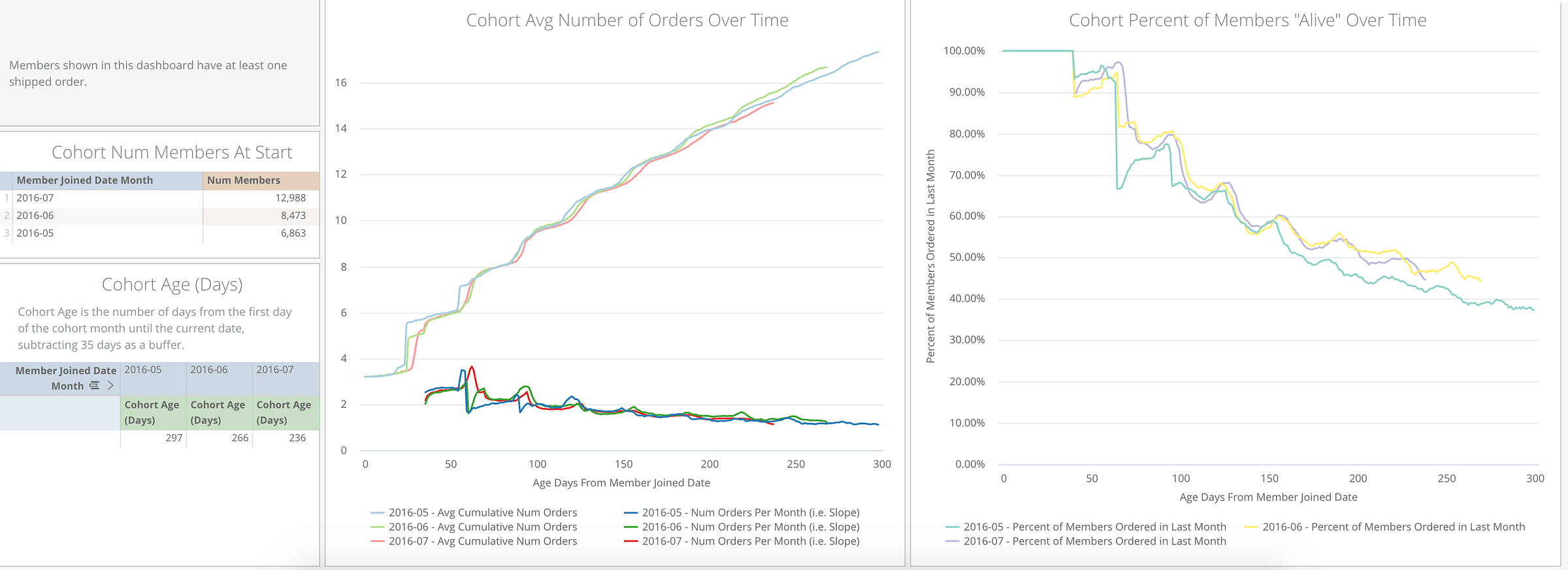Cohorts Dashboard

Some tiles that we find crucial to our dashboard are:

1. To compare how many orders were placed by members across different cohorts.
2. To track the percent of members that have ordered in the last month over time as a metric for how engaged a cohort is.

We also want to list how many members start in each cohort, as well as how long we follow them for.

#### 1. Preparing the Data

a. Create a table that gets each members start date (or any other metric for which you want to begin tracking members from)

• This table will have two variables: member ID and the start date

b. Create a cross-joined table

• Create a table with all dates (starting from the earliest member start date) up until today’s date
• Create a table with all distinct member IDs
• Cross join the two above tables so that you have a row for every date-member ID combination
• Left join the table created in step 1a on member ID.
• Create a new column which will calculate the number of days from that members start date to the date in that row.

This can be achieved in SQL using code like:

``CASE WHEN DATEDIFF(days, member_join_date::date, date) >= 0 THEN DATEDIFF(days, member_join_date::date, date) ELSE NULL END as age_days_from_member_join_date``
• Optional: Delete rows where the number of days is null, as there’s no value in seeing what happens for a member before they joined.

This will leave you with a table looking something like this:

c. Join in any relevant additional information

• Left join in additional tables that contain information that can be connected with a date.
• In this example, an orders table will be joined onto the cross joined table. However, any information that you want to track over time can be joined in, such as log in dates, or financial information.
``LEFT JOIN orders ON (cross_joined_table.member_id = orders.member_id AND cross_joined_table.date = orders.created_date)``
• To create our ‘Cohort Percent of Members Alive Over Time’ tile, we need to know, at each row, whether a member has ordered within the last month. The below code will create a column that gets the date of the current row (if there is an order on that day, and if not, it returns the order date of that members previous order.
``COALESCE(orders.order_date, lag(orders.order_date ignore nulls) over (PARTITION BY cross_joined_table.member_id ORDER BY cross_joined_table.date, orders.id)) AS last_order_date``
• After the last order date has been calculated for each row, you can then create a binary column to determine if a member has ordered in the last month.
``CASE WHEN DATEDIFF(day, last_order_date, date) <= 30 THEN 1 ELSE 0 END AS ordered_in_last_4_weeks``

Now you have the necessary data to create a Looker cohorts dashboard!

#### 2. Preparing the LookML

a. Creating the Cohorts view

• When creating the Looker view for Cohorts, the primary key should be the date concatenated to the member ID.
• Looker Measures such as number of members (to calculate cohort size), number of orders (to calculate how much each member contributes to a cohort) should use a count_distinct calculation type. Number of members ordered in last 4 weeks (to calculate how active a cohort is) should use a sum_distinct calculation type. All of these measures should use the primary key as the sql_distinct_key.

#### 3. Creating the Cohorts Dashboard

The four tiles that will be created for this Cohorts Dashboard will be:

• Cohort Num Members At Start
• Cohort Age (Days)
• Cohort Avg Number of Orders Over Time
• Cohort Percent of Members “Alive” Over Time

a. Cohort Num Members At Start

This is the simplest tile to create on the Cohorts Dashboard. It is a table that shows how many members belong to that cohort at day 0.

Filters:

• Age Days from Member Joined Date = 0

Dimensions:

• Member Joined Month

Measures:

• Num Members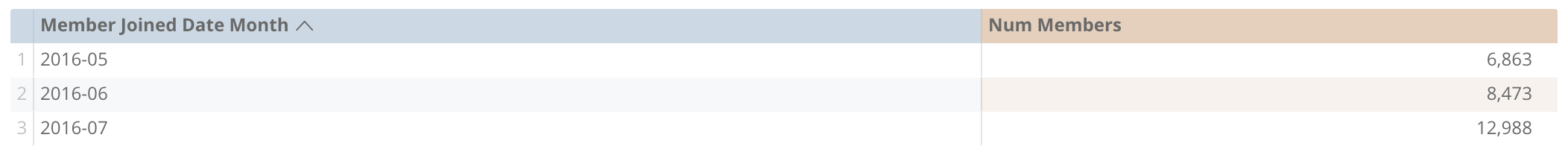Number of Members in Cohort

b. Cohort Age (Days)

This tile will show how long you are following each cohort for.

Pivots:

• Member Joined Month

Dimensions:

• Age Days from Member Joined Date. This will be hidden from the visualization.

Table Calculations:

• Under Cohort Max Age:

When following a cohort through time, we want to make sure we only follow them up until they have data (which is typically present day), and we also want to make sure that all members are followed for the same duration. E.g. For an October Cohort, if a member joined in October 31st, we want to follow that member for the same duration as a member that joined on October 1st.

To do this, we create a table calculation that returns True if the number of days from member join date is less than the number of days from the member start month until now minus 35 days. The 35 days is to ensure that we follow all members in each month cohort for the same number of days. This will be hidden from the visualization.

This is a very important concept that is used across many tiles in this Cohorts Dashboard. This table calculation will be hidden from the visualization.

``\${cohorts.age_days_from_member_join_date} <= diff_days(\${member_start_dates.member_join_month}, now()) - 35``
• First or Last Row or Cohort:

The eventual output for this tile is to display the value in the last row for a cohort (i.e. the last day you follow that cohort for). However, because that value is on a different row for each cohort, it isn’t straightforward to retrieve it in Looker. This table calculation will return the value in the row if it is the first or last row for that cohort. The reason we also retrieve the first row in the cohort is to safeguard against nulls, because if there is a null in a row, Looker does not display that row in your Dashboard. There will be nulls if a user is analyzing at least one cohort which has an age of 0. This table calculation will be hidden from the visualization.

``if(((offset(\${under_cohort_max_age}, 1) = no AND \${under_cohort_max_age} = yes) OR offset(\${under_cohort_max_age}, -1) = no AND \${under_cohort_max_age} = yes) , \${cohorts.age_days_from_member_joined_date}, null)``
• Cohort Age (Days)

This retrieves the maximum value from the first_or_last_row_of_cohort table calculation, which should be the amount of days that the cohort is followed for.

``max(offset_list(\${first_or_last_row_of_cohort},0 , 5000))``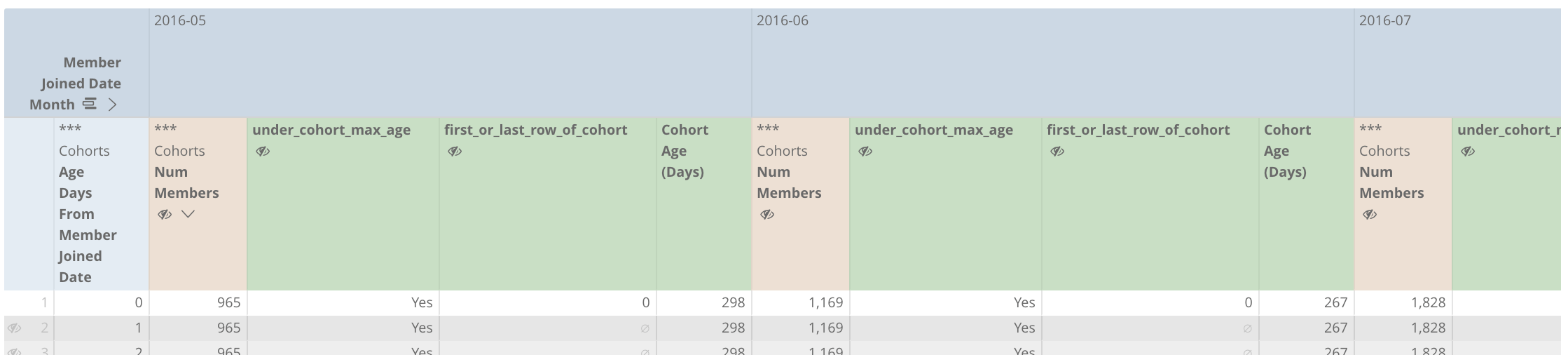Cohort Age

In the visualization, you will also want to select ‘Limit Displayed Rows’ and only show the first row, as this is the only information that is needed.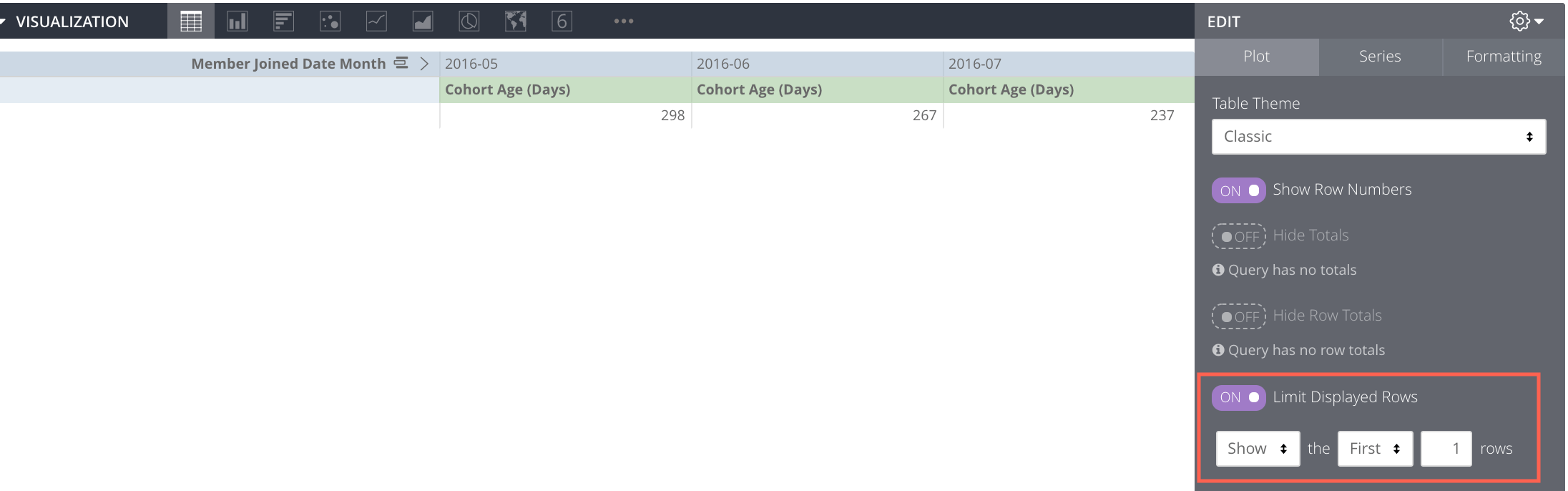Cohort Age Visualization

c. Cohort Avg Number of Orders Over Time

This tile will show how your members are ordering from each cohort over time. It also gives some insight into the frequency at which a typical member in a cohort orders.

Pivots:

• Member Joined Month

Dimensions:

• Age Days from Member Joined Date

Measures:

• Number of Members. This will be hidden from the visualization.
• Number of Orders. This will be hidden from the visualization.

Table Calculations:

• Cumulative Orders:
``running_total(\${cohorts.num_orders})``

This will be hidden from the visualization.

• Under Cohort Max Age

This is the same concept that was illustrated in 3b.

• Average Cumulative Orders:

If the row is under the cohort max age, then divide the total number of orders by the number of members in the cohort, otherwise return null.

``if(\${under_cohort_max_age}=yes, round(\${cumulative_total_revenue}/\${cohorts.num_members},2), null)``
• Orders Per 4.5 Weeks (i.e. Slope):

This table calculation will return the slope of the average cumulative orders table calculation over the past 4.5 weeks.

``offset(\${avg_cumulative_orders},-1) - offset(\${avg_cumulative_orders}, -35) + (\${avg_cumulative_orders} - offset(\${avg_cumulative_orders},-1))/2``
• Show X Axis

This will return Yes as long as one of the pivots returns Yes for “Under Cohort Max Age”. You can then right click on this table calculation and select “Hide No’s from Visualization”. This in conjunction with deselecting the option to “Plot Null Values” in Looker can force Looker graphs to dynamically not plot rows for cohorts that are under the cohort max age, while still plotting those rows for other applicable cohorts. This is highlighted in the image below.

``if(\${cohorts.age_days_from_member_join_date} <= max(pivot_row(max(if(\${under_cohort_max_age} = yes, \${cohorts.age_days_from_member_join_date}, null)))), yes, no)``

Using these steps, you can achieve a visualization like below: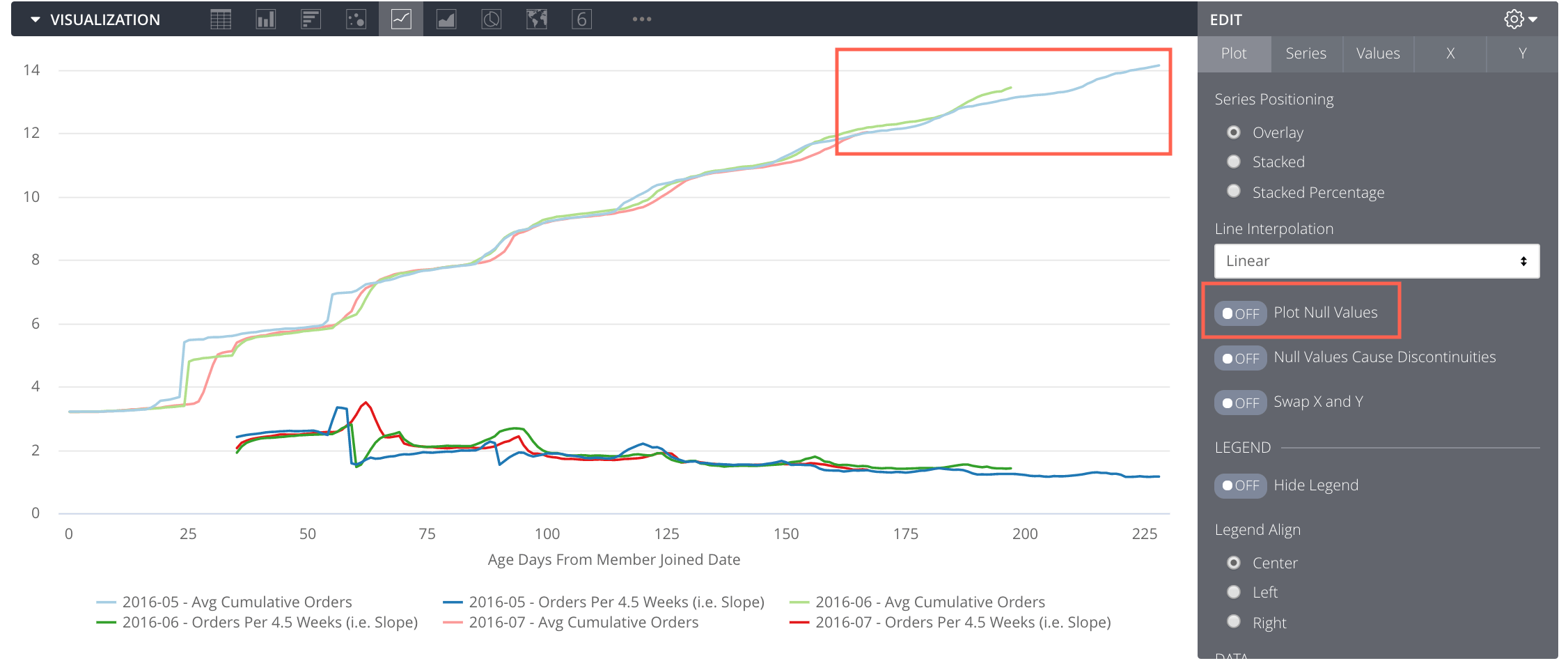Avg Number of Orders Per Members Over Time

d. Cohort Percent of Members “Alive” Over Time

This tile shows how active (or “alive”) your cohort members are over time, and gives some insight into cohort retention, by calculating what percent of them have placed an order in the last 4 weeks.

Pivots:

• Member Joined Month

Dimensions:

• Age Days from Member Joined Date

Measures:

• Number of Members. This will be hidden from the visualization.
• Num Ordered in Last 4 Weeks. This will be hidden from the visualization.

Table Calculations:

• Under Cohort Max Age:

This is the same concept that was illustrated in 3b.

• Percent of Members Ordered in Last 4 Weeks:

If the row is under the cohort max age, then divide the total number of members who ordered in the past 4 weeks by the number of members in the cohort, otherwise return null.

``if(\${under_cohort_max_age}=yes, \${cohorts.num_ordered_in_last_4_weeks}/ max(\${cohorts.num_members}), null)``
• Show X Axis

This is the same concept that was illustrated in 3c.

Using these steps, you can achieve a visualization like below: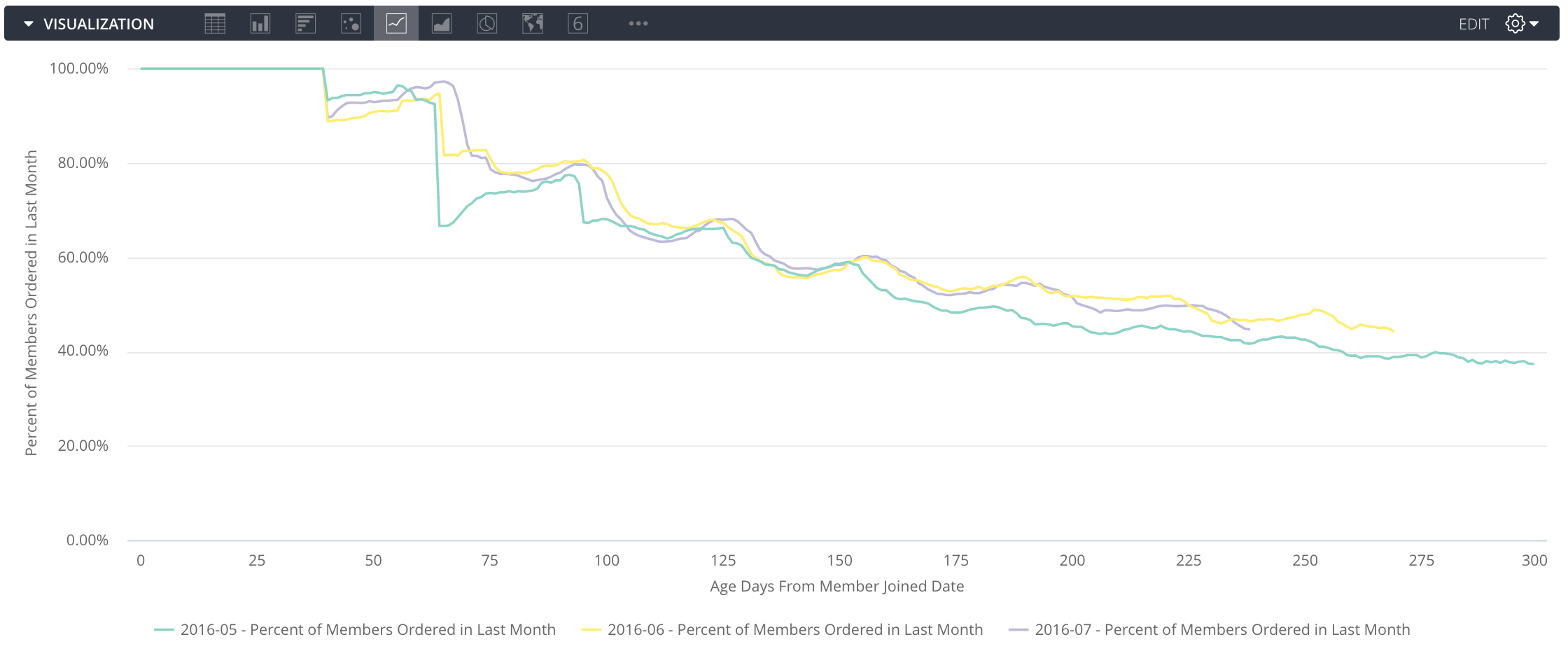Percent of Members “Alive” Over Time

### 4. Potential Additions

Dashboard-wide filters can also be used to enhance the effectiveness of your Cohorts Dashboard. These filters will typically be a first attribute for a member, such as a members first product bought, or how a member was introduced to your business. These filters can provide a further way to slice your cohorts, and supplement your analyses.